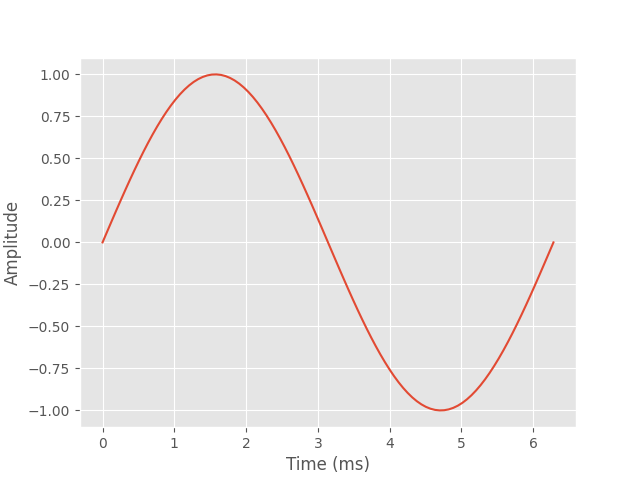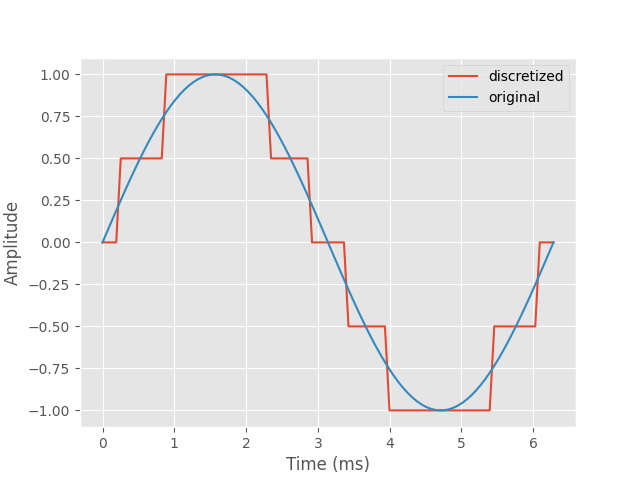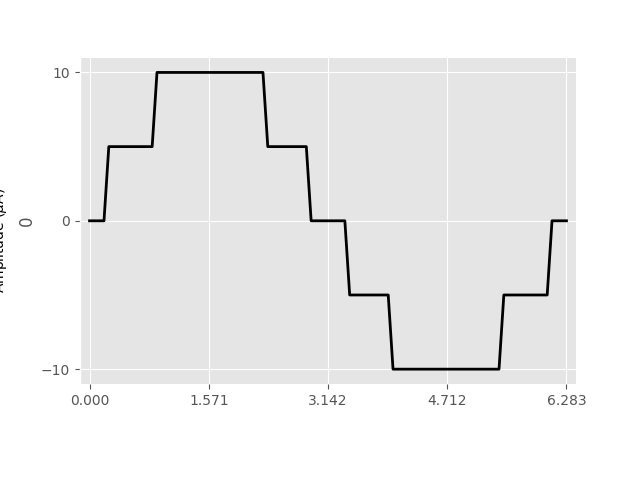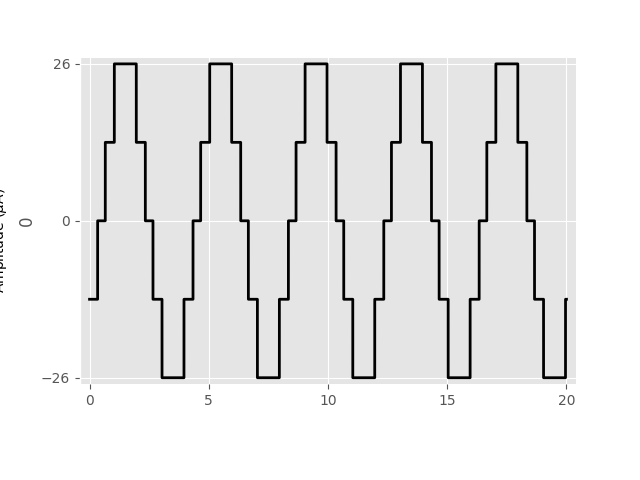# Generating a sinusoidal pulse train¶

This example shows how to build a sinusoidal pulse train from scratch.

In addition to built-in stimuli such as BiphasicPulse and BiphasicPulseTrain, you can also create your own Stimulus by manually specifying the values for the data container and time axis.

Consider a sine wave:

from pulse2percept.utils.constants import DT
from pulse2percept.stimuli import Stimulus
import numpy as np
import matplotlib.pyplot as plt
plt.style.use('ggplot')

t = np.linspace(0, 2 * np.pi, 100)
x = np.sin(t)
plt.plot(t, x)
plt.xlabel('Time (ms)')
plt.ylabel('Amplitude')Out:

Text(22.347222222222214, 0.5, 'Amplitude')


To turn this sine wave into a stimulus feasible for a retinal implant, it will have to be discretized to a number of different amplitude levels.

The following code turns the sine wave into 5 different amplitude levels:

levels = np.linspace(-1, 1, num=5)
data = levels[np.argmin(np.abs(x[:, np.newaxis] - levels), axis=1)]

plt.plot(t, data, label='discretized')
plt.plot(t, x, label='original')
plt.xlabel('Time (ms)')
plt.ylabel('Amplitude')
plt.legend()Out:

<matplotlib.legend.Legend object at 0x7fbff32dee90>


We can turn this signal into a Stimulus object as follows:

stim = Stimulus(10 * data.reshape((1, -1)), time=t)
stim.plot()Out:

<AxesSubplot:ylabel='0'>


Alternatively, we can automate this process by creating a new class SinusoidalPulse that inherits from Stimulus:

class SinusoidalPulse(Stimulus):

def __init__(self, amp, freq, phase, stim_dur, n_levels=5, dt=DT):
"""Sinusoidal pulse

Parameters
----------
amp : float
Maximum stimulus amplitude (uA)
freq : float
Ordinary frequency of the sine wave (Hz)
phase : float
Phase of the sine wave (rad)
stim_dur : float
Stimulus duration (ms)
n_levels : int, optional, default: 5
Number of discretization levels
dt : float, optional, default: 0.001
Smallest time step (ms)
"""
# Create the sine wave:
t = np.arange(0, stim_dur + dt / 2, dt)
x = np.sin(2 * np.pi * freq * t + phase)
# Discretize it:
levels = np.linspace(-1, 1, num=n_levels)
data = levels[np.argmin(np.abs(x[:, np.newaxis] - levels), axis=1)]
# Reshape data to 1xM so it is interpreted as M data points for a
# single electrode:
data = amp * data.reshape((1, -1))
# Call the Stimulus constructor:
super(SinusoidalPulse, self).__init__(data, time=t, compress=True)


Then we can create a new pulse as follows:

sine = SinusoidalPulse(26, 0.25, -np.pi / 4, 20)
sine.plot()Out:

<AxesSubplot:ylabel='0'>


Total running time of the script: ( 0 minutes 0.373 seconds)

Gallery generated by Sphinx-Gallery